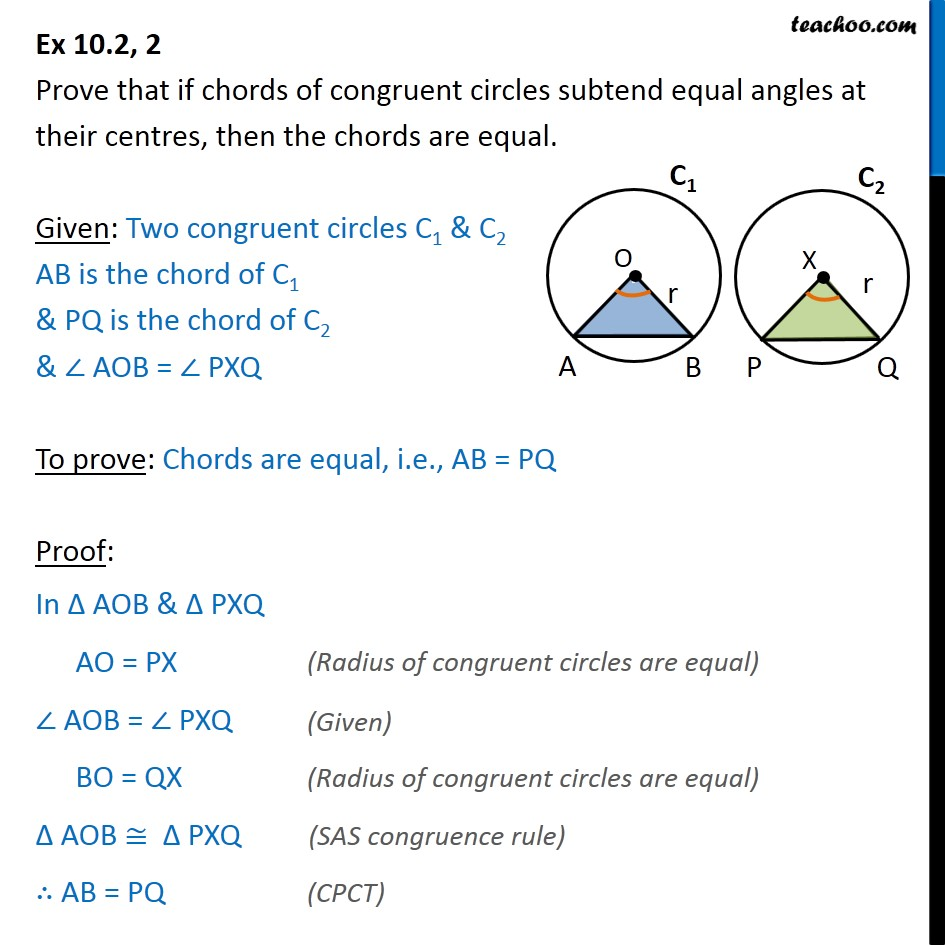Ex 9.1

Chapter 9 Class 9 Circles
Serial order wiseLearn in your speed, with individual attention - Teachoo Maths 1-on-1 Class

### Transcript

Ex 9.1, 2 Prove that if chords of congruent circles subtend equal angles at their centres, then the chords are equal. Given: Two congruent circles C1 & C2 AB is the chord of C1 & PQ is the chord of C2 & AOB = PXQ To prove: Chords are equal, i.e., AB = PQ Proof: In AOB & PXQ AO = PX AOB = PXQ BO = QX AOB PXQ AB = PQ